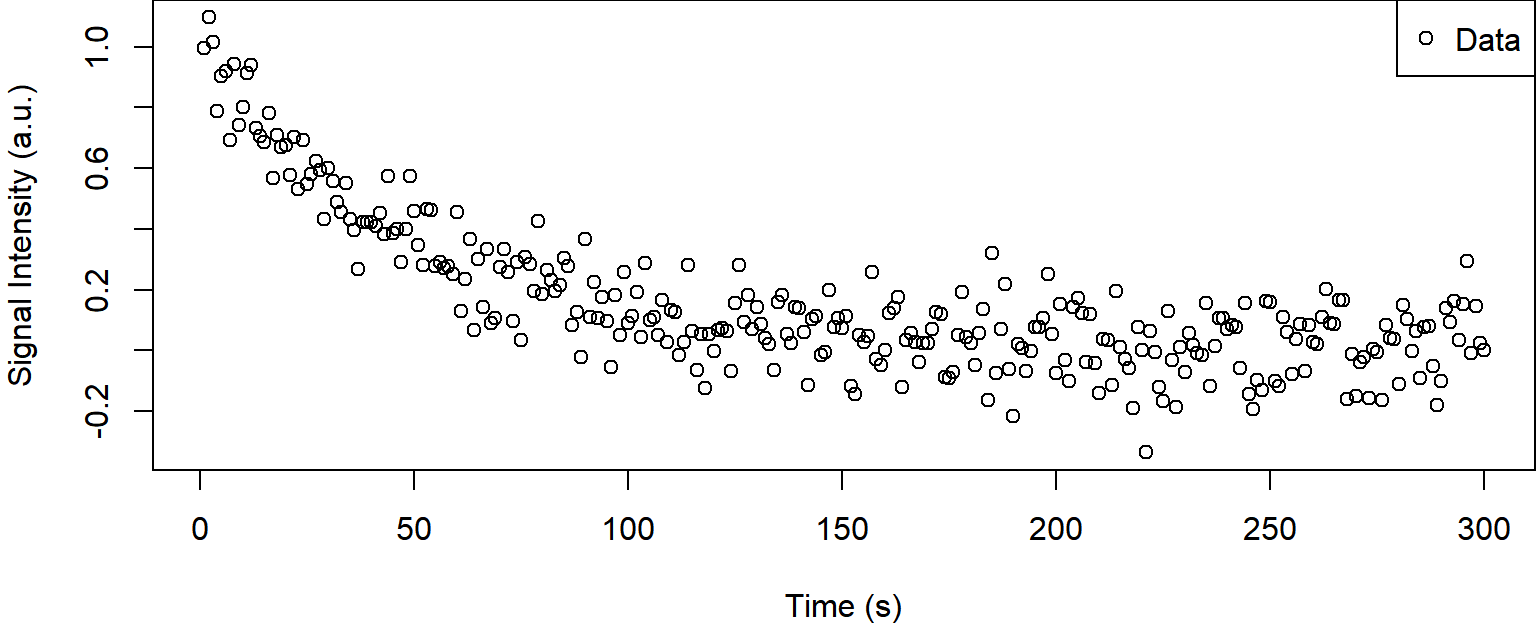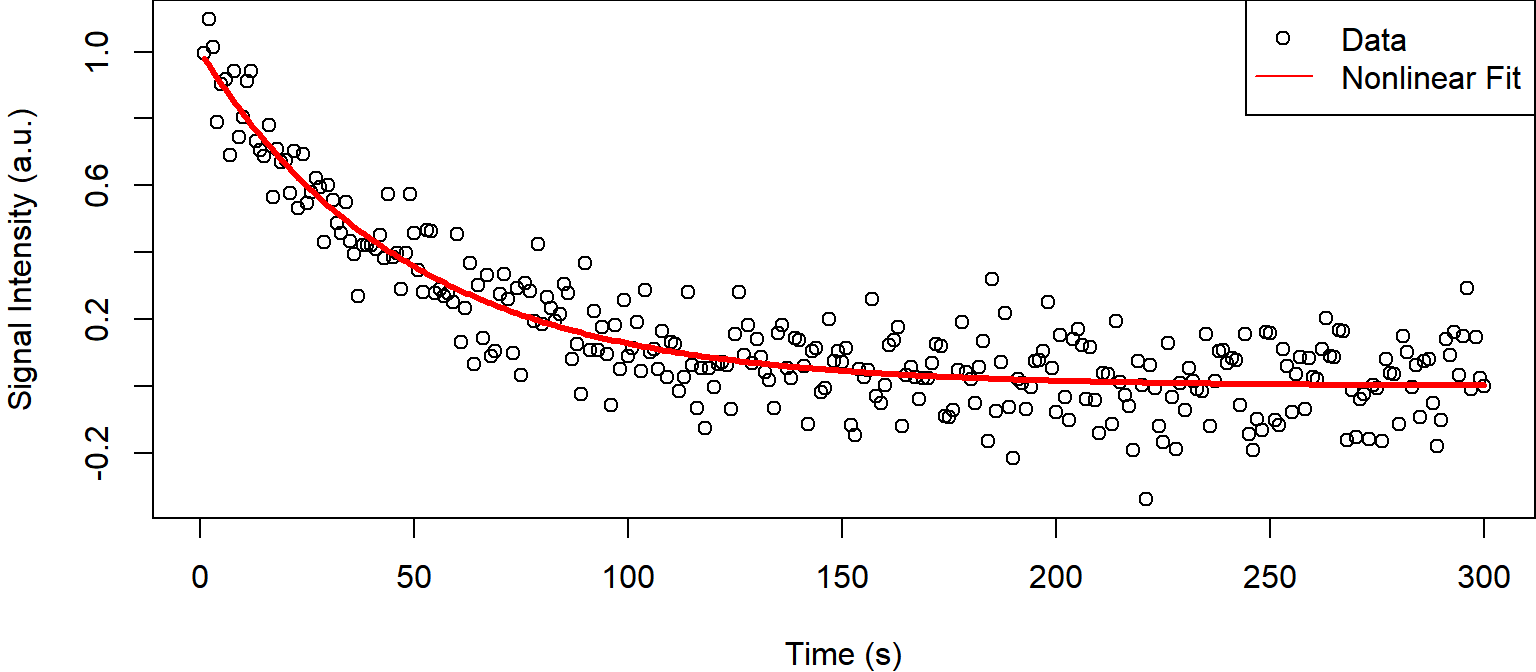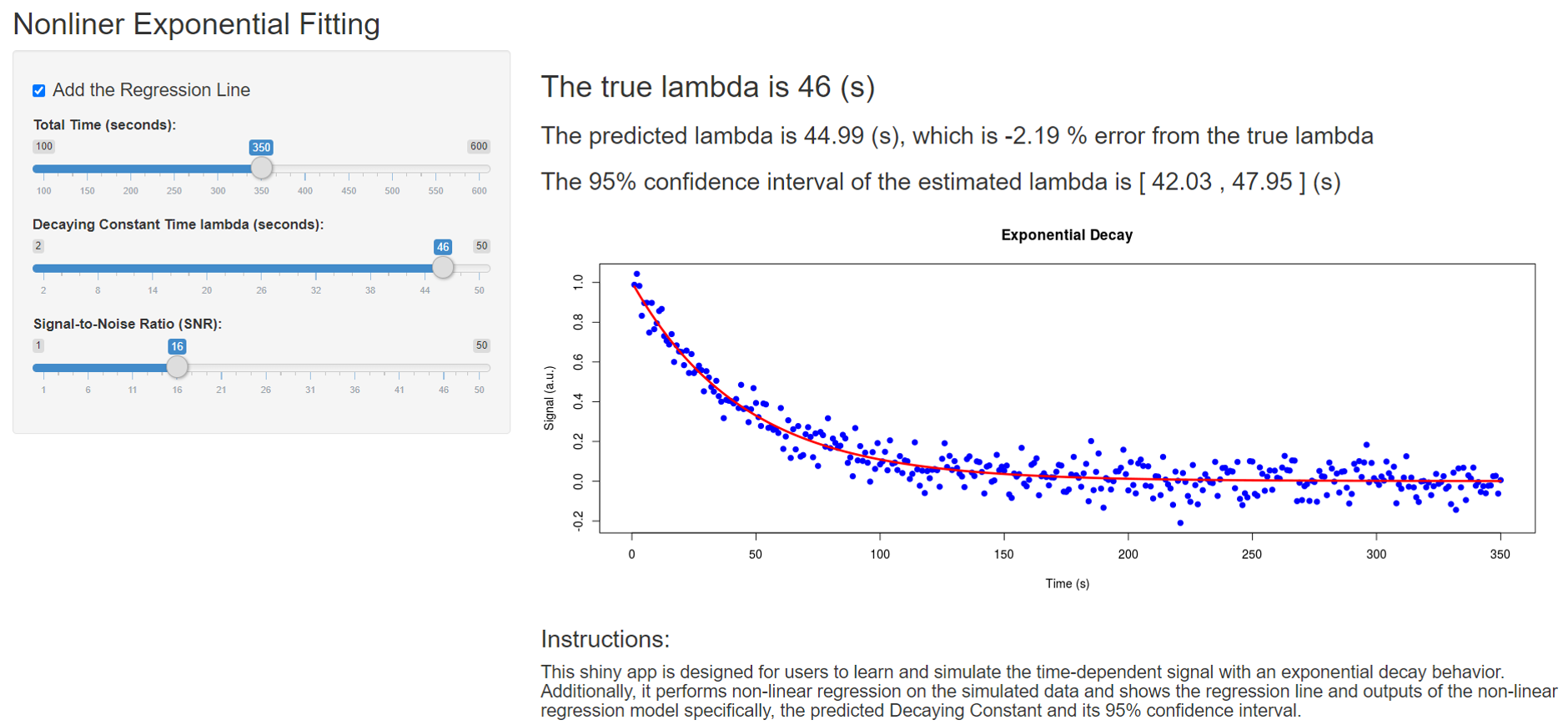# R Shiny Application: Nonlinear Exponential Fit

## Introduction

• Exponential decay is a simple mathematical model yet very effective in modeling real-world signal behavior in many areas of Natural Science such as Radioactivity, Vibrations, Chemical Reactions, Geophysics, and etc.

• This simple Shiny App was developed for users who want to learn about the exponential decay model, its key parameters, and how the key parameters alter the signal.

• Additionally, the Shiny App enables the users to show prediction and its 95% confidence interval estimated from the nonlinear regression model.

## Exponential Decay Model

Normalized signal equation is written in the following form:

$S(t) = exp(-\frac{t}{\lambda}) + \epsilon$

where $$S(t)$$ is the normalized signal intensity (a.u.), $$t$$ is time (s), $$\lambda$$ is the Decaying Time Constant (s), and $$\epsilon$$ is random noise.

## Simulated Data

set.seed(1234567); t<-seq(1,300); lambda<-50 # true lambda
s<-exp(-t/lambda)+0.1*rnorm(length(t)); par(mar=c(4,4,0,0))
plot(t,s, xlab="Time (s)", ylab = "Signal Intensity (a.u.)")
legend("topright", legend=c("Data"), pch = c(1))## Non-Linear Exponential Fitting

df<-data.frame(t = t, s = s); par(mar=c(4,4,0,0))
fit<-nls(s ~ exp(-t/lambda), data = df,start = list(lambda=35.0))
sfit<-predict(fit,list(t=df\$t))
plot(t,s, xlab="Time (s)", ylab = "Signal Intensity (a.u.)", col="black")
lines(t,sfit,lwd=3,col="red")
legend("topright", legend=c("Data", "Nonlinear Fit"), col=c("black", "red"), pch=c(1,-1), lty=c(0,1))## Prediction and Relative Error

paste("True lambda: ",lambda," (s)")
##  "True lambda:  50  (s)"
paste("Predicted lambda: ",round(coef(fit)[],digits=2))
##  "Predicted lambda:  48.64"
paste("Relative Error: ",round(100*(coef(fit)[]-lambda)/lambda),"%")
##  "Relative Error:  -3 %"

## A Simple R Shiny Apps

Here is the weblink to a very simple R shiny app that performs the nonlinear exponential fitting.